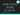# How dates work in Excel

August 29, 2017

If you’re going to do any date manipulation, it’s important to understand how dates work in Excel. They can be a little confusing at first, but you’ll quickly see that it’s not as complicated as you might think. We’ll also discuss what “serial numbers” are when you use an Excel date function. Let’s get started.

## Understanding Excel Dates

Behind the scenes, a date in Excel is simply a number with special formatting.

Open up a new worksheet in Excel and type in the following date:

``1/1/1900``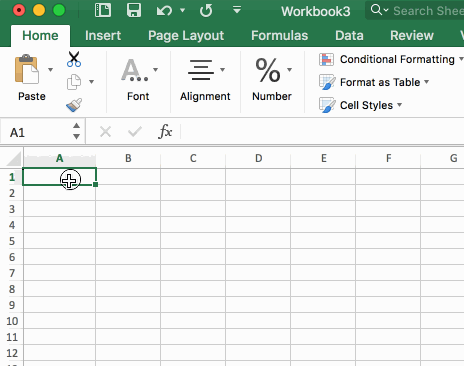When you see the result, you may think that this date represents `1/1/2000` since it has the short format of `1/1/00`. A quick inspection of the formula in the formula bar will show you the true date of `1/1/1900`.

Now, change the format to a number.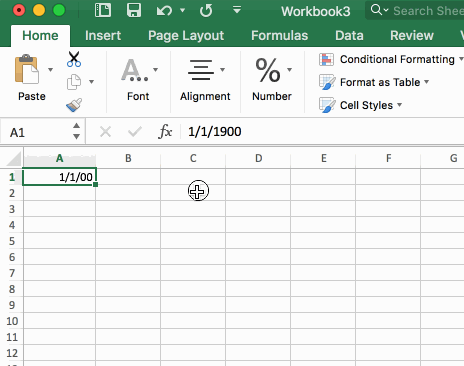Interestingly enough, turning `1/1/1900` to a number result in the number `1`.

How come?

The reason is because Excel dates are just numbers with special formatting. Each whole number represents one day, starting on `1/1/1900`. Day #2 is `1/2/1900`.

Excel dates are just numbers with special formatting.

### What about the time? How is that calculated?

If Excel dates are “just a number,” then how is time calculated?

The way Excel handles time is that time is a percentage of a 24 hour period. Meaning that `100% = 24 hours`. And `50% = 12 hours`.

Take a look at the following table. I’ve created a special number format to make this easier to understand:

And if you’re interested in the special number format I used, here it is:

``m/d/yyyy | hh"h" mm"m" ss"s";@``

Notice the `1.50` number in cell `A7`? The `.50` portion is stating “half of the day” which would be noon, or 12pm.

Another way to think about this is that 12 hours out of 24 hours is 1/2 (`12 / 24 = 0.50`).

#### What fraction of a number is needed to get a single minute?

To understand how to get the “number version” (so-to-say) of an Excel date for a single minute, we can do some simple math.

Let’s take the number `1.5` and look at Excel’s equivalent date:

``1.5 = 1/1/1900 | 12h 00m 00s``

How could we add 1 second to this date if we used a number?

That `0.50` part represents 12 hours. Let’s convert that to minutes.

``12 hours * 60 min / hour = 720 minutes``

Also, let’s note that `24 hours = 1440 minutes`.

``720 minutes / 1440 minutes = 0.50``

If we added `1` to `720` and did the calculation again:

``721 minutes / 1440 minutes = 0.500694444444444``

So let’s plug in:

``1.500694444444444``

Into an Excel cell and convert it to a date.

#### What fraction of a number is needed to get a single second?

It’s the same story here as with getting a single minute (above).

Convert 24 hours to seconds:

``24 hours * 60 min/hour * 60 sec/min = 86,400 seconds``

Going back to the example of `1.5`:

``1.5 = 1/1/1900 | 12h 00m 00s``

We’ll figure out how to add a second to this number.

The `0.5` portion, again, represents 12 hours, but that also represented 720 minutes, and we can also think about this in seconds, which would be 43,200 seconds.

Add 1 second to `43,200` and determine what fraction that is out of `86,400` seconds:

``43201 / 86400 = 0.500011574074074``

Putting that back into Excel as `1.500011574074074`:## Excel Dates in Formulas

All this talk about numbers and I almost forgot to mention how Excel uses dates in functions. Whenever you use a date function in Excel, you may notice that Excel asks for a `serial_number`, what’s with that?

### What is a Serial Number?

A serial number is simply the decimal representation of a date in Excel. This is the same number that we have been dealing with in the above sections.

What you may not realize, though, is that when you add a cell reference like `A1` to an Excel date function like `YEAR()`, you’re actually handing the `YEAR()` function the `serial_number`, even if `A1` is formatted as a date.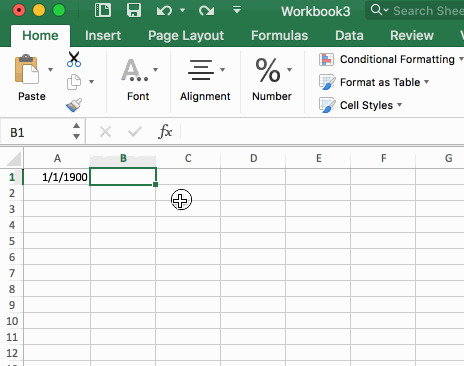A quick inspection of the function (using the `F9` key) will show that cell `A1` gets evaluated to its decimal equivalent, or `1` in this case: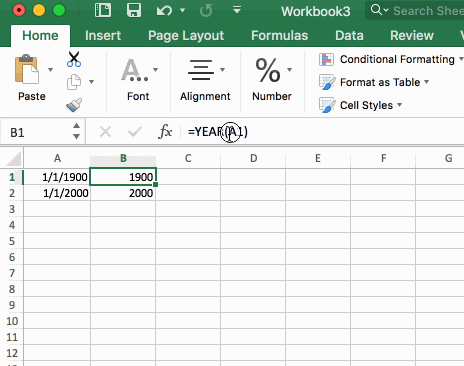Wow, you read the whole article! You know, people who make it this far are true learners. And clearly, you value learning. Would you like to learn more about Excel? Please consider supporting me by buying me a coffee (it takes a lot of coffee to write these articles!).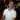Written by Joseph who loves teaching about Excel.